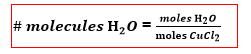# Problem: A hydrate of copper (II) chloride has the following formula: CuCl2 • xH2 O. The water in a 3.41-g sample of the hydrate was driven off by heating. The remaining sample had a mass of 2.69 g .Find the number of waters of hydration (x) in the hydrate.

###### FREE Expert Solution

Recall that for hydrates, water molecules can be evaporated by heating.

We can solve for x = waters of hydration or the # of molecules of H2O in the hydrate by doing these steps:

1. Determine mass of water removed
2. Do mass to mole conversion for both H2O and CuCl2.
3. Determine x or the # of H2O molecules using the equation:88% (318 ratings)###### Problem Details

A hydrate of copper (II) chloride has the following formula: CuCl2 • xH2 O. The water in a 3.41-g sample of the hydrate was driven off by heating. The remaining sample had a mass of 2.69 g .

Find the number of waters of hydration (x) in the hydrate.